Share

# HC Verma solutions for Class 12 Concepts of Physics 1 chapter 18 - Geometrical Optics [Latest edition]

Textbook page

#### Chapters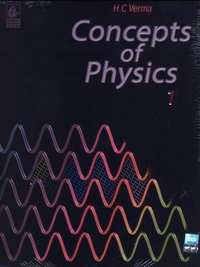## Chapter 18: Geometrical Optics

### HC Verma solutions for Class 12 Concepts of Physics 1 Chapter 18 Geometrical Optics Short Answers [Page 410]

Short Answers | Q 1 | Page 410

Is the formula "Real depth/Apparent depth = μ" valid if viewed from a position quite away from the normal?

Short Answers | Q 2 | Page 410

Can you ever have a situation in which a light ray goes undeviated through a prism?

Short Answers | Q 3 | Page 410

Why does a diamond shine more than a glass piece cut to the same shape?

Short Answers | Q 4 | Page 410

A narrow beam of light passes through a slab obliquely and is then received by an eye following figure. The index of refraction of the material in the slab fluctuates slowly with time. How will it appear to the eye? The twinkling of stars has a similar explanation.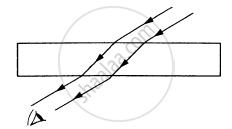Short Answers | Q 5 | Page 410

Can a plane mirror ever form a real image?

Short Answers | Q 6 | Page 410

If a piece of paper is placed at the position of a virtual image of a strong light source, will the paper burn after sufficient time? What happens if the image is real? What happens if the image is real but the source is virtual?

Short Answers | Q 7 | Page 410

Can a virtual image be photographed by a camera?

Short Answers | Q 8 | Page 410

In motor vehicles, a convex mirror is attached near the driver's seat to give him the view of the traffic behind. What is the special function of this convex mirror which a plane mirror can not do?

Short Answers | Q 9 | Page 410

If an object far away from a convex mirror moves towards the mirror, the image also moves. Does it move faster, slower or at the same speed as compared to the object?

Short Answers | Q 10 | Page 410

Suppose you are inside the water in a swimming pool near an edge. A friends is standing on the edge. Do you find your friend taller or shorter than his usual height?

Short Answers | Q 11 | Page 410

The equation of refraction at a spherical surface is $\frac{\mu_2}{\nu} - \frac{\mu_1}{\mu} = \frac{\mu_2 - \mu_1}{R}$
Taking $R = \infty$ show that this equation leads to the equation
$\frac{\text{ Real depth }}{\text{ Apparent depth }} = \frac{\mu_2}{\mu_1}$
for refraction at a plane surface.

Short Answers | Q 12 | Page 410

A thin converging lens is formed with one surface convex and the other plane. Does the position of image depend on whether the convex surface or the plane surface faces the object?

Short Answers | Q 13 | Page 410

A single lens is mounted in a tube. A parallel beam enters the tube and emerges out of the tube as a divergent beam. Can you say with certainty that there is a diverging lens in the tube?

Short Answers | Q 14 | Page 410

An air bubble is formed inside water. Does it act as a converging lens or a diverging lens?

Short Answers | Q 15 | Page 410

Two converging lenses of unequal focal lengths can be used to reduce the aperture of a parallel beam of light without loosing the energy of the light. This increase the intensity. Describe how the converging lenses should be placed to do this.

Short Answers | Q 16 | Page 410

If a spherical mirror is dipped in water, does its focal length change?

Short Answers | Q 17 | Page 410

If a thin lens is dipped in water, does its focal length change?

Short Answers | Q 18 | Page 410

Can mirrors give rise to chromatic aberration?

Short Answers | Q 19 | Page 410

A laser light is focussed by a converging lens. Will there be a significant chromatic aberration?

### HC Verma solutions for Class 12 Concepts of Physics 1 Chapter 18 Geometrical Optics MCQ [Pages 410 - 411]

MCQ | Q 1 | Page 410

A point source of light is placed in front of a plane mirror.

• All the reflected rays meet at a point when produced backward.

•  Only the reflected rays close to the normal meet at a point when produced backward.

•  Only the reflected rays making a small angle with the mirror meet at a point when produced backward.

• Light of different colours make different images.

MCQ | Q 2 | Page 411

Total internal reflection can take place only if

• light goes from optically rarer medium (smaller refractive index) to optically denser medium

• light goes from optically denser medium to rarer
medium

• the refractive indices of the two media are close to each other

• the refractive indices of the two media are widely different.

MCQ | Q 3 | Page 411

In image formation from spherical mirrors, only paraxial rays are considered because they

•  are easy to handle geometrically

• contain most of the intensity of the incident light

• from nearly a point image of a point source

• show minimum dispersion effect.

MCQ | Q 4 | Page 411

A point object is placed at a distance of 30 cm from a convex mirror of focal length 30 cm. The image will form at

•  infinity

• pole

• focus

• 15 cm behind the mirror.

MCQ | Q 5 | Page 411

following Figure shows two rays A and B being reflected by a mirror and going as A' and B'. The mirror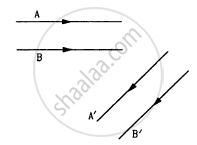• is plane

• is convex

• is concave

• may be any spherical mirror.

MCQ | Q 6 | Page 411

The image formed by a concave mirror

• is always real

•  is always virtual

• is certainly real if the object is virtual

•  is certainly virtual if the object is real.

MCQ | Q 7 | Page 411

Following figure  shows three transparent media of refractive indices $\mu_1 , \mu_2 \text{ and } \mu_3$.  A point object O is placed in the medium $\mu_2$.  If the entire medium on the right of the spherical surface has refractive index  $\mu_3$, the image forms at O". In the situation shown,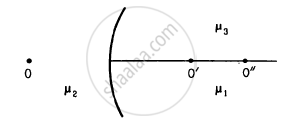• the image forms between O' and O"

• the image forms to the left of O'

• the image forms to the right of O"

• two images form, one at O' and the other at O".

MCQ | Q 8 | Page 411

Four modifications are suggested in the lens formula to include the effect of the thickness t of the lens. Which one is likely to be correct?

• $\frac{1}{\nu} - \frac{1}{u} = \frac{t}{uf}$

• $\frac{t}{\nu^2} - \frac{1}{u} = \frac{1}{f}$

• $\frac{1}{\nu - t} - \frac{1}{u + t} = \frac{1}{f}$

• $\frac{1}{\nu} - \frac{1}{u} + \frac{t}{uv} = \frac{t}{f}$

MCQ | Q 9 | Page 411

A double convex lens has two surfaces of equal radii R and refractive index $m = 1 \cdot 5$

• $f = R/2$

• $f = R$
• $f = - R$

• $f = 2R$

MCQ | Q 10 | Page 411

A point source of light is placed at a distance of 2 f from a converging lens of focal length f. The intensity on the other side of the lens is maximum at a distance

• f

• between f and 2 f

• f

• more than 2 f.

MCQ | Q 11 | Page 411

A parallel beam of light is incident on a converging lens parallel to its principal axis. As one moves away from the lens on the other side on its principal axis, the intensity of light

• remains constant

•  continuously increases

• continuously decreases

• first increases then decreases.

MCQ | Q 12 | Page 411

A symmetric double convex lens in cut in two equal parts by a plane perpendicular to the principal axis. If the power of the original lens was 4 D, the power a cut-lens will be

• 2 D

• 3 D

• 4 D

• 5 D.

MCQ | Q 13 | Page 411

A symmetric double convex lens is cut in two equal parts by a plane containing the principal axis. If the power of the original lens was 4 D, the power of a divided lens will be

•  2 D

• 3 D

• 4 D

• 5 D.

MCQ | Q 14 | Page 411

Two concave lenses L1 and L2 are kept in contact with each other. If the space between the two lenses is filled with a material of smaller refractive index, the magnitude of the focal length of the combination

•  becomes undefined

• remains unchanged

• increases

• decreases.

MCQ | Q 15 | Page 411

A thin lens is made with a material having refractive index
$\mu = 1 \cdot 5$. Both the side are convex. It is dipped in water $\mu = 1 \cdot 33$. It will behave like

• a convergent lens

• a divergent lens

•  a rectangular slab

• a prism.

MCQ | Q 16 | Page 411

A convex lens is made of a material having refractive index
$1 \cdot 2$ Both the surfaces of the lens are convex. If it is dipped into water (μ = 1.33), it will behave like

•  a convergent lens

• a divergent lens

• a rectangular slab

•  a prism.

MCQ | Q 17 | Page 411

A point object O is placed on the principal axis of a convex lens of focal length f = 20 cm at a distance of 40 cm to the left of it. The diameter of the lens is 10 cm. An eye is placed 60 cm to right of the lens and a distance h below the principal axis. The maximum value of h to see the image is

• 0

• 2.5 cm

• 5 cm

• 10 cm.

MCQ | Q 18 | Page 411

The rays of different colours fail to converge at a point after going through a converging lens. This defect is called

•  spherical aberration

• distortion

•  coma

• chromatic aberration.

### HC Verma solutions for Class 12 Concepts of Physics 1 Chapter 18 Geometrical Optics MCQ [Page 412]

MCQ | Q 1 | Page 412

If the light moving in a straight line bends by a small but fixed angle, it may be a case of
(a) reflection
(b) refraction
(c) diffraction
(d) dispersion.

MCQ | Q 2 | Page 412

Mark the correct options.

•  If the incident rays are converging, we have a real object.

• If the final rays are converging, we have a real image.

• The image of a virtual object is called a virtual image.

• If the image is virtual, the corresponding object is called a virtual object.

MCQ | Q 3 | Page 412

Which of the following (referred to a spherical mirror) do (does) not depend on whether the rays are paraxial or not?
(a) Pole
(b) Focus
(d) Principal axis

MCQ | Q 4 | Page 412

The image of an extended object, placed perpendicular to the principal axis of a mirror, will be erect if
(a) the object and the image are both real
(b) the object and the image are both virtual
(c) the object is real but the image is virtual
(d) the object is virtual but the image is real.

MCQ | Q 5 | Page 412

A convex lens forms a real image of a point object placed on its principals axis. If the upper half of the lens is painted black,
(a) the image will be shifted downward
(b) the image will be shifted upward
(c) the image will not be shifted
(d) the intensity of the image will decrease.

MCQ | Q 6 | Page 412

Consider three converging lenses L1, L2 and L3 having identical geometrical construction. The index of refraction of L1 and L2 are $\mu_1 \text{ and } \mu_2$ respectively. The upper half of the lens L3 has a refractive index $\mu_1$ and the lower half has $\mu_2$  following figure . A point object O is imaged at O1 by the lens L1 and at O2 by the lens L2placed in same position. If L3 is placed at the same place,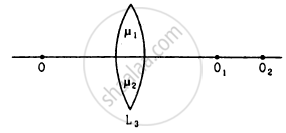(a) there will be an image at O1
(b) there will be an image at O2.
(c) the only image will form somewhere between O1 and O2
(d) the only image will form away from O2.

MCQ | Q 7 | Page 412

A screen is placed a distance 40 cm away from an illuminated object. A converging lens is placed between the source and the screen and its is attempted to form the image of the source on the screen. If no position could be found, the focal length of the lens

• must be less than 10 cm

• must be greater than 20 cm

• must not be greater than 20 cm

• must not be less than 10 cm.

### HC Verma solutions for Class 12 Concepts of Physics 1 Chapter 18 Geometrical Optics 19 [Pages 412 - 417]

Q 1 | Page 412

A concave mirror having a radius of curvature 40 cm is placed in front of an illuminated point source at a distance of 30 cm from it. Find the location of the image.

Q 2 | Page 412

A concave mirror forms an image of 20 cm high object on a screen placed 5.0 m away from the mirror. The height of the image is 50 cm. Find the focal length of the mirror and the distance between the mirror and the object.

Q 3 | Page 412

A concave mirror has a focal length of 20 cm. Find the position or positions of an object for which the image-size is double of the object-size.

Q 4 | Page 412

A 1 cm object is placed perpendicular to the principal axis of a convex mirror of focal length 7.5 cm. Find its distance from the mirror if the image formed is 0.6 cm in size.

Q 5 | Page 412

A candle flame 1.6 cm high is imaged in a ball bearing of diameter 0.4 cm. If the ball bearing is 20 cm away from the flame, find the location and the height of the image.

Q 6 | Page 412

A 3 cm tall object is placed at a distance of 7.5 cm from a convex mirror of focal length 6 cm. Find the location, size and nature of the image.

Q 7 | Page 412

A U-shaped wire is placed before a concave mirror having radius of curvature 20 cm as shown in figure. Find the total length of the image.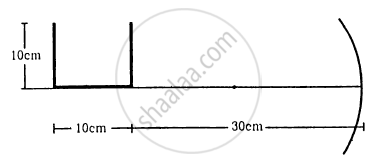Q 8 | Page 412

A man uses a concave mirror for shaving. He keeps his face at a distance of 25 cm from the mirror and gets an image which is 1.4 times enlarged. Find the focal length of the mirror.

Q 9 | Page 413

Find the diameter of the image of the moon formed by a spherical concave mirror of focal length 7.6 m. The diameter of the moon is 3450 km and the distance between the earth and the moon is 3.8 × 105 km.

Q 10 | Page 413

A particle goes in a circle of radius 2.0 cm. A concave mirror of focal length 20 cm is placed with its principal axis passing through the centre of the circle and perpendicular to its plane. The distance between the pole of the mirror and the centre of the circle is 30 cm. Calculate the radius of the circle formed by the image.

Q 11 | Page 413

A concave mirror of radius R is kept on a horizontal table (See figure). Water (refractive index = μ) is poured into it up to a height h. Where should an object be placed so that its image is formed on itself?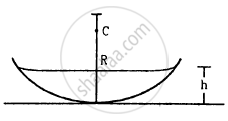Q 12 | Page 413

A point source S is placed midway between two converging mirrors having equal focal length f as shown in figure. Find the values of d for which only one image is formed.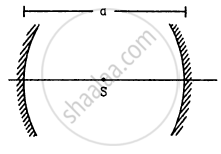Q 13 | Page 413

A converging mirror M1, a point source S and a diverging mirror M2 are arranged as shown in figure. The source is placed at a distance of 30 cm from M1. The focal length of each of the mirrors is 20 cm. Consider only the images formed by a maximum of two reflections. It is found that one image is formed on the source itself. (a) Find the distance between the two mirrors. (b) Find the location of the image formed by the single reflection from M2.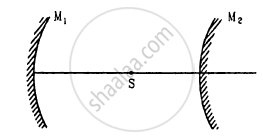Q 14 | Page 413

A light ray falling at an angle of 45° with the surface of a clean slab of ice of thickness 1.00 m is refracted into it at an angle of 30°. Calculate the time taken by the light rays to cross the slab. Speed of light in vacuum = 3 × 108 m s−1.

Q 15 | Page 413

A pole of length 1.00 m stands half dipped in a swimming pool with water level 50.0 cm higher than the bed. The refractive index of water is 1.33 and sunlight is coming at an angle of 45° with the vertical. Find the length of the shadow of the pole on the bed.

Q 16 | Page 413

A small piece of wood is floating on the surface of a 2.5 m deep lake. Where does the shadow form on the bottom when the sum is just setting? Refractive index of water = 4/3.

Q 17 | Page 413

An object P is focussed by a microscope MA glass slab of thickness 2.1 cm is introduced between P and M. If the refractive index of the slab is 1.5, by what distance should the microscope be shifted to focus the object again?

Q 18 | Page 413

A vessel contains water up to a height of 20 cm and above it an oil up to another 20 cm. The refractive indices of the water and the oil are 1.33 and 1.30 respectively. Find the apparent depth of the vessel when viewed from above.

Ex. 19 | Q 19 | Page 413

Locate the image of the point P as seen by the eye in the figure.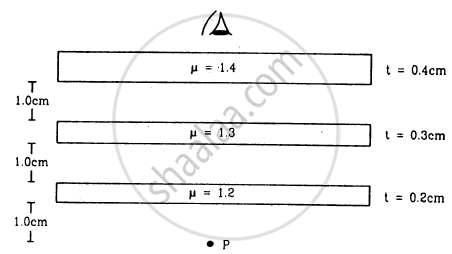Q 20 | Page 413

k transparent slabs are arranged one over another. The refractive indices of the slabs are μ1, μ2, μ3, ... μk and the thicknesses are t1 t2t3, ... tk. An object is seen through this combination with nearly perpendicular light. Find the equivalent refractive index of the system which will allow the image to be formed at the same place.

Q 21 | Page 413

A cylindrical vessel of diameter 12 cm contains 800π cm3 of water. A cylindrical glass piece of diameter 8.0 cm and height 8.0 cm is placed in the vessel. If the bottom of the vessel under the glass piece is seen by the paraxial rays (see figure), locate its image. The index of refraction of glass is 1.50 and that of water is 1.33.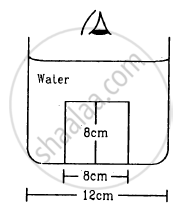Q 22 | Page 413

Consider the situation in figure. The bottom of the pot is a reflecting plane mirror, S is a small fish and T is a human eye. Refractive index of water is μ. (a) At what distance(s) from itself will the fish see the image(s) of the eye? (b) At what distance(s) from itself will the eye see the image(s) of the fish.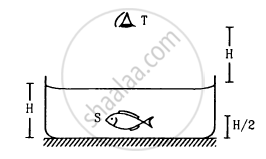Q 23 | Page 413

A small object is placed at the centre of the bottom of a cylindrical vessel of radius 3 cm and height 4 cm filled completely with water. Consider the ray leaving the vessel through a corner. Suppose this ray and the ray along the axis of the vessel are used to trace the image. Find the apparent depth of the image and the ratio of real depth to the apparent depth under the assumptions taken. Refractive index of water = 1.33.

Q 24 | Page 414

A cylindrical vessel, whose diameter and height both are equal to 30 cm, is placed on a horizontal surface and a small particle P is placed in it at a distance of 5.0 cm from the centre. An eye is placed at a position such that the edge of the bottom is just visible (see figure). The particle P is in the plane of drawing. Up to what minimum height should water be poured in the vessel to make the particle P visible?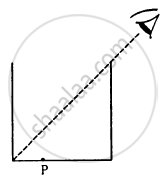Q 25 | Page 414

A light ray is incident at an angle of 45° with the normal to a √2 cm thick plate (μ = 2.0). Find the shift in the path of the light as it emerges out from the plate.

Q 26 | Page 414

An optical fibre (μ = 1.72) is surrounded by a glass coating (μ = 1.50). Find the critical angle for total internal reflection at the fibre-glass interface.

Q 27 | Page 414

A light ray is incident normally on the face AB of a right-angled prism ABC (μ = 1.50) as shown in figure. What is the largest angle ϕ for which the light ray is totally reflected at the surface AC?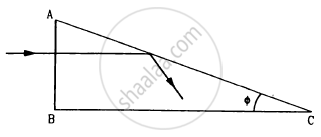Q 28 | Page 414

Find the maximum angle of refraction when a light ray is refracted from glass (μ = 1.50) to air.

Q 29 | Page 414

Light is incident from glass (μ = 1.5) to air. Sketch the variation of the angle of deviation δ with the angle of incident i for 0 < i < 90°.

Q 30 | Page 414

Light is incident from glass (μ = 1.50) to water (μ = 1.33). Find the range of the angle of deviation for which there are two angles of incidence.

Q 31 | Page 414

Light falls from glass (μ = 1.5) to air. Find the angle of incidence for which the angle of deviation is 90°.

Q 32 | Page 414

A point source is placed at a depth h below the surface of water (refractive index = μ). (a) Show that light escapes through a circular area on the water surface with its centre directly above the point source. (b) Find the angle subtended by a radius of the area on the source.

Q 33 | Page 414

A container contains water up to a height of 20 cm and there is a point source at the centre of the bottom of the container. A rubber ring of radius r floats centrally on the water. The ceiling of the room is 2.0 m above the water surface. (a) Find the radius of the shadow of the ring formed on the ceiling if r = 15 cm. (b) Find the maximum value of r for which the shadow of the ring is formed on the ceiling. Refractive index of water = 4/3.

Q 35 | Page 414

Find the angle of minimum deviation for an equilateral prism made of a material of refractive index 1.732. What is the angle of incidence for this deviation?

Q 35 | Page 414

Find the angle of deviation suffered by the light ray shown in figure. The refractive index μ = 1.5 for the prism material.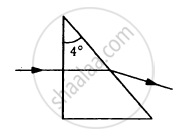Q 36 | Page 414

A light ray, going through a prism with the angle of prism 60°, is found to deviate by 30°. What limit on the refractive index can be put from these data?

Q 37 | Page 414

Locate the image formed by refraction in the situation shown in figure.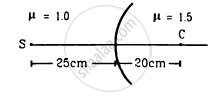Q 38 | Page 414

A spherical surface of radius 30 cm separates two transparent media and B with refractive indices 1.33 and 1.48 respectively. The medium A is on the convex side of the surface. Where should a point object be placed in medium A so that the paraxial rays become parallel after refraction at the surface?

Q 39 | Page 415

Figure shows a transparent hemisphere of radius 3.0 cm made of a material of refractive index 2.0. (a) A narrow beam of parallel rays is incident on the hemisphere as shown in the figure. Are the rays totally reflected at the plane surface? (b) Find the image formed by the refraction at the first surface. (c) Find the image formed by the reflection or by the refraction at the plane surface. (d) Trace qualitatively the final rays as they come out of the hemisphere.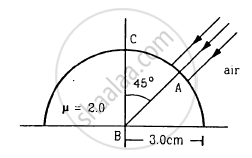Q 40 | Page 415

A small object is embedded in a glass sphere (μ = 1.5) of radius 5.0 cm at a distance 1.5 cm left to the centre. Locate the image of the object as seen by an observer standing (a) to the left of the sphere and (b) to the right of the sphere.

Q 41 | Page 415

A biconvex thick lens is constructed with glass (μ = 1.50). Each of the surfaces has a radius of 10 cm and the thickness at the middle is 5 cm. Locate the image of an object placed far away from the lens.

Q 42 | Page 415

A narrow pencil of parallel light is incident normally on a solid transparent sphere of radius r. What should be the refractive index is the pencil is to be focussed (a) at the surface of the sphere, (b) at the centre of the sphere.

Q 43 | Page 415

One end of a cylindrical glass rod (μ = 1.5) of radius 1.0 cm is rounded in the shape of a hemisphere. The rod is immersed in water (μ = 4/3) and an object is placed in the water along the axis of the rod at a distance of 8.0 cm from the rounded edge. Locate the image of the object.

Q 44 | Page 415

A paperweight in the form of a hemisphere of radius 3.0 cm is used to hold down a printed page. An observer looks at the page vertically through the paperweight. At what height above the page will the printed letters near the centre appear to the observer?

Q 45 | Page 415

Solve the previous problem if the paperweight is inverted at its place so that the spherical surface touches the paper.

Q 46 | Page 415

A hemispherical portion of the surface of a solid glass sphere (μ = 1.5) of radius r is silvered to make the inner side reflecting. An object is placed on the axis of the hemisphere at a distance 3r from the centre of the sphere. The light from the object is refracted at the unsilvered part, then reflected from the silvered part and again refracted at the unsilvered part. Locate the final image formed.

Q 47 | Page 415

The convex surface of a thin concavo-convex lens of glass of refractive index 1.5 has a radius of curvature 20 cm. The concave surface has a radius of curvature 60 cm. The convex side is silvered and placed on a horizontal surface as shown in figure. (a) Where should a pin be placed on the axis so that its image is formed at the same place? (b) If the concave part is filled with water (μ = 4/3), find the distance through which the pin should be moved so that the image of the pin again coincides with the pin.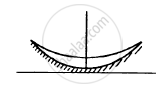Q 48 | Page 415

A double convex lens has focal length 25 cm. The radius of curvature of one of the surfaces is double of the other. Find the radii, if the refractive index of the material of the lens is 1.5.

Q 49 | Page 415

The radii of curvature of a lens are + 20 cm and + 30 cm. The material of the lens has a refracting index 1.6. Find the focal length of the lens (a) if it is placed in air, and (b) if it is placed in water (μ = 1.33).

Q 50 | Page 415

Lenses are constructed by a material of refractive index 1.50. The magnitude of the radii of curvature are 20 cm and 30 cm. Find the focal lengths of the possible lenses with the above specifications.

Q 51 | Page 415

A thin lens made of a material of refractive index μ2 has a medium of refractive index μ1on one side and a medium of refractive index μ3 on the other side. The lens is biconvex and the two radii of curvature have equal magnitude RA beam of light travelling parallel to the principal axis is incident on the lens. Where will the image be formed if the beam is incident from (a) the medium μ1 and (b) from the medium μ3?

Q 52 | Page 415

A convex lens has a focal length of 10 cm. Find the location and nature of the image if a point object is placed on the principal axis at a distance of (a) 9.8 cm, (b) 10.2 cm from the lens.

Q 53 | Page 415

A slide projector has to project a 35 mm slide (35 mm × 23 mm) on a 2 m × 2 m screen at a distance of 10 m from the lens. What should be the focal length of the lens in the projector?

Q 54 | Page 415

A particle executes a simple harmonic motion of amplitude 1.0 cm along the principal axis of a convex lens of focal length 12 cm. The mean position of oscillation is at 20 cm from the lens. Find the amplitude of oscillation of the image of the particle.

Q 55 | Page 415

An extended object is placed at a distance of 5.0 cm from a convex lens of focal length 8.0 cm. (a) Draw the ray diagram (to the scale) to locate the image and from this, measure the distance of the image from the lens. (b) Find the position of the image from the lens formula and see how close the drawing is to the correct result.

Q 56 | Page 415

A pin of length 2.00 cm is placed perpendicular to the principal axis of a converging lens. An inverted image of size 1.00 cm is formed at a distance of 40.0 cm from the pin. Find the focal length of the lens and its distance from the pin.

Q 57 | Page 416

A convex lens produces a double size real image when an object is placed at a distance of 18 cm from it. Where should the object be placed to produce a triple size real image?

Q 58 | Page 416

A pin of length 2.0 cm lies along the principal axis of a converging lens, the centre being at a distance of 11 cm from the lens. The focal length of the lens is 6 cm. Find the size of the image.

Q 59 | Page 416

The diameter of the sun is 1.4 × 109 m and its distance from the earth is 1.5 × 1011 m. Find the radius of the image of the sun formed by a lens of focal length 20 cm.

Q 60 | Page 416

A 5.0 diopter lens forms a virtual image which is 4 times the object placed perpendicularly on the principal axis of the lens. Find the distance of the object from the lens.

Q 61 | Page 416

A diverging lens of focal length 20 cm and a converging mirror of focal length 10 cm are placed coaxially at a separation of 5 cm. Where should an object be placed so that a real image is formed at the object itself?

Q 62 | Page 416

A converging lens of focal length 12 cm and a diverging mirror of focal length 7.5 cm are placed 5.0 cm apart with their principal axes coinciding. Where should an object be placed so that its image falls on itself?

Q 63 | Page 416

A converging lens and a diverging mirror are placed at a separation of 15 cm. The focal length of the lens is 25 cm and that of the mirror is 40 cm. Where should a point source be placed between the lens and the mirror so that the light, after getting reflected by the mirror and then getting transmitted by the lens, comes out parallel to the principal axis?

Q 64 | Page 416

A converging lens of focal length 15 cm and a converging mirror of focal length 10 cm are placed 50 cm apart with common principal axis. A point source is placed in between the lens and the mirror at a distance of 40 cm from the lens. Find the locations of the two images formed.

Q 65 | Page 416

Consider the situation described in the previous problem. Where should a point source be placed on the principal axis so that the two images form at the same place?

Q 66 | Page 416

A converging lens of focal length 15 cm and a converging mirror of focal length 10 cm are placed 50 cm apart. If a pin of length 2.0 cm is placed 30 cm from the lens farther away from the mirror, where will the final image form and what will be the size of the final image?

Q 67 | Page 416

A point object is placed on the principal axis of a convex lens (f = 15 cm) at a distance of 30 cm from it. A glass plate (μ = 1.50) of thickness 1 cm is placed on the other side of the lens perpendicular to the axis. Locate the image of the point object.

Q 68 | Page 416

A convex lens of focal length 20 cm and a concave lens of focal length 10 cm are placed 10 cm apart with their principal axes coinciding. A beam of light travelling parallel to the principal axis and having a beam diameter 5.0 mm, is incident on the combination. Show that the emergent beam is parallel to the incident one. Find the beam diameter of the emergent beam.

Q 69 | Page 416

A diverging lens of focal length 20 cm and a converging lens of focal length 30 cm are placed 15 cm apart with their principal axes coinciding. Where should an object be placed on the principal axis so that its image is formed at infinity?

Q 70 | Page 416

A 5 mm high pin is placed at a distance of 15 cm from a convex lens of focal length 10 cm. A second lens of focal length 5 cm is placed 40 cm from the first lens and 55 cm from the pin. Find (a) the position of the final image, (b) its nature and (c) its size.

Q 71 | Page 416

A point object is placed at a distance of 15 cm from a convex lens. The image is formed on the other side at a distance of 30 cm from the lens. When a concave lens is placed in contact with the convex lens, the image shifts away further by 30 cm. Calculate the focal lengths of the two lenses.

Q 72 | Page 416

Two convex lenses, each of focal length 10 cm, are placed at a separation of 15 cm with their principal axes coinciding. (a) Show that a light beam coming parallel to the principal axis diverges as it comes out of the lens system. (b) Find the location of the virtual image formed by the lens system of an object placed far away. (c) Find the focal length of the equivalent lens. (Note that the sign of the focal length is positive although the lens system actually diverges a parallel beam incident on it.)

Q 73 | Page 416

A ball is kept at a height h above the surface of a heavy transparent sphere made of a material of refractive index μ. The radius of the sphere is R. At t = 0, the ball is dropped to fall normally on the sphere. Find the speed of the image formed as a function of time for $t < \sqrt{\frac{2h}{g}}$ . Consider only the image by a single refraction.

Q 74 | Page 416

A particle is moving at a constant speed V from a large distance towards a concave mirror of radius R along its principal axis. Find the speed of the image formed by the mirror as a function of the distance x of the particle from the mirror.

Q 75 | Page 416

A small block of mass m and a concave mirror of radius R fitted with a stand lie on a smooth horizontal table with a separation d between them. The mirror together with its stand has a mass m. The block is pushed at t = 0 towards the mirror so that it starts moving towards the mirror at a constant speed V and collides with it. The collision is perfectly elastic. Find the velocity of the image (a) at a time t < d/V, (b) at a time t > d/V.

Q 76 | Page 416

A gun of mass M fires a bullet of mass m with a horizontal speed V. The gun is fitted with a concave mirror of focal length f facing towards the receding bullet. Find the speed of separation of the bullet and the image just after the gun was fired.

Q 77 | Page 416

A mass m = 50 g is dropped on a vertical spring of spring constant 500 N m−1 from a height h = 10 cm as shown in figure. The mass sticks to the spring and executes simple harmonic oscillations after that. A concave mirror of focal length 12 cm facing the mass is fixed with its principal axis coinciding with the line of motion of the mass, its pole being at a distance of 30 cm from the free end of the spring. Find the length in which the image of the mass oscillates.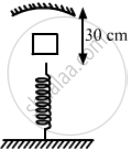Q 78 | Page 417

Two concave mirrors of equal radii of curvature R are fixed on a stand facing opposite directions. The whole system has a mass m and is kept on a frictionless horizontal table following figure. Two blocks A and B, each of mass m, are placed on the two sides of the stand. At t = 0, the separation between A and the mirrors is 2 R and also the separation between B and the mirrors is 2 R. The block B moves towards the mirror at a speed v. All collisions which take place are elastic. Taking the original position of the mirrors-stand system to be x = 0 and X-axis along AB, find the position of the images of A and B at t = (a) R/v  (b)  3R/v (c) 5R/v.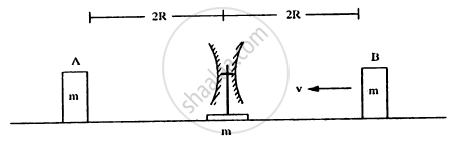Q 79 | Page 417

Consider the situation shown in figure. The elevator is going up with an acceleration of 2.00 m s−2 and the focal length of the mirror is 12.0 cm. All the surfaces are smooth and the pulley is light. The mass-pulley system is released from rest (with respect to the elevator) at t = 0 when the distance of B from the mirror is 42.0 cm. Find the distance between the image of the block B and the mirror at t = 0.200 s. Take g = 10 m s−2.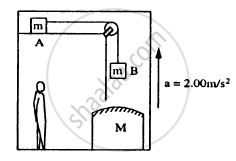## Chapter 18: Geometrical Optics## HC Verma solutions for Class 12 Concepts of Physics 1 chapter 18 - Geometrical Optics

HC Verma solutions for Class 12 Concepts of Physics 1 chapter 18 (Geometrical Optics) include all questions with solution and detail explanation. This will clear students doubts about any question and improve application skills while preparing for board exams. The detailed, step-by-step solutions will help you understand the concepts better and clear your confusions, if any. Shaalaa.com has the CBSE Class 12 Concepts of Physics 1 solutions in a manner that help students grasp basic concepts better and faster.

Further, we at Shaalaa.com provide such solutions so that students can prepare for written exams. HC Verma textbook solutions can be a core help for self-study and acts as a perfect self-help guidance for students.

Concepts covered in Class 12 Concepts of Physics 1 chapter 18 Geometrical Optics are Light Process and Photometry, Total Internal Reflection, Refraction, Ray Optics - Mirror Formula, Reflection of Light by Spherical Mirrors, Refraction Through a Prism, Refraction by a Lens, Snell’s Law, Concave Mirror, Rarer and Denser Medium, Lensmaker's Formula, Thin Lens Formula, Lenses, The Microscope, Some Natural Phenomena Due to Sunlight, Dispersion by a Prism, Combination of Thin Lenses in Contact, Telescope, The Eye, Power of a Lens, Magnification, Refraction at Spherical Surfaces.

Using HC Verma Class 12 solutions Geometrical Optics exercise by students are an easy way to prepare for the exams, as they involve solutions arranged chapter-wise also page wise. The questions involved in HC Verma Solutions are important questions that can be asked in the final exam. Maximum students of CBSE Class 12 prefer HC Verma Textbook Solutions to score more in exam.

Get the free view of chapter 18 Geometrical Optics Class 12 extra questions for Class 12 Concepts of Physics 1 and can use Shaalaa.com to keep it handy for your exam preparation